Name:    9HQuiz31

Multiple Choice
Identify the choice that best completes the statement or answers the question.

1.

What is the distance between the two points A(-5,7) and B(3,10).
 a.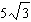c.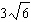b.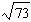d.2.

What is the slope between the two points A(3,7) B(8,5)
 a. 0.75 c. -2 b. 0.5 d. -0.5

3.

What is the midpoint between the two points E(3,6) and F(11,-24)
 a.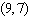c. (7,-9) b. (-9,-7) d. (9,-9)

4.

The points (-5,v) and (-2,w) are on a line parallel to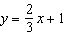.  What is the value of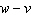?
 a. -2 c.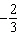b. 2 d.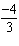5.

A line intersects the positive x-axis and positive y-axis as shown.  Which of the following is a possible equation of this line?a.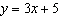c.b.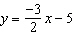d.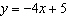6.

The point (p,q) is one the line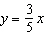as shown.  If the area of the rectangle is 105, then what is the value of “p”?a. 21 c. 7 b. 35 d. 28

7.

Given that the coordinates (m,n) lie on the line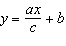.  Which of the following coordinates would also be on the line?
 a.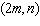c.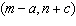b.d.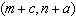8.

Which of the following graph has a slope of zero?
 a.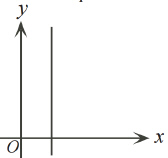c.b.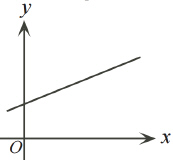d.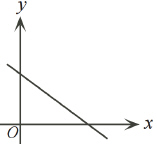9.

Given that the points A(1,2), B(4,3), and C(-2,-3) are three vertices of a parallelogram.  Which of the following can be a coordinate of a fourth vertex?
 a. (3,-1) c. (8,7) b. (-7,-4) d. (2,-2)

10.

If “x” and “y” are positive integers with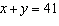, then the largest possible value of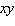is?
 a. 450 c. 420 b. 418 d. 482